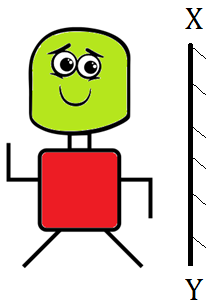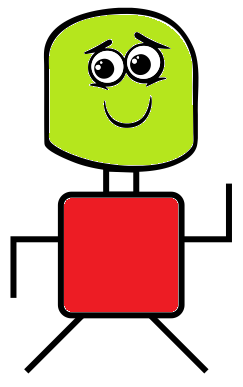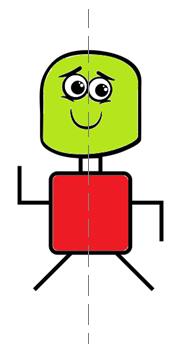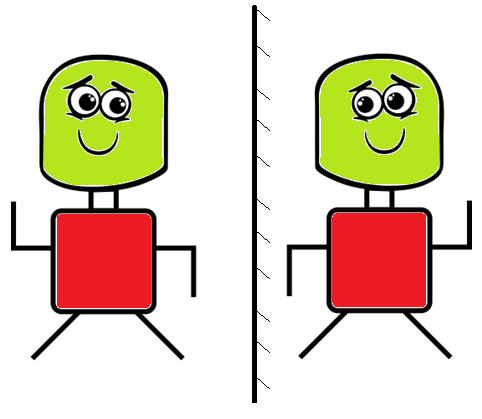Question of The Day28-10-2019

What will be the mirror image of the given figure about the XY axis?Correct Answer : b )Explanation :

To find the mirror image of any object, we have to consider the following rules/points:

• The Left-hand side portion of the image appears on the right side in the mirror image while the right-hand side portion of the image appears on the left-hand side in the mirror image.
• The portion of the image which is bilaterally symmetrical, will appear the same in the mirror image.

The mirror image of the given figure can be explained by the movement of the eyes and hands of the given figure.In the given image if we divide the given image into two halves by a vertical axis passing through the center of the body, then the hand on the left side of the image will appear in the right side of the mirror image while the hand on the right side of the image will appear on the left side of the mirror image. Also, the left eye in the image will appear as the right eye and the right eye in the image will appear as the left eye.

So, the mirror image of the given figure will beHence, (B) is the correct answer.

Such type of question is easy to score and is asked in exams like SSC CGL, SSC MTS, SSC CPO,  SSC CHSL, RRB JE, RRB NTPC, RRB GROUP D, etc. Try and attempt free mock tests at PendulumEdu. One can also clear all exam related doubts through PendulumEdu’s one-to-one session.0